# RSA 算法笔记

## 数学概念

### 同余定理

ab(modp)

373(mod17) $37 \equiv 3 \pmod{17}$
522(mod17) $5 \equiv 22\pmod{17}$

$\equiv$ = $=$ 非常相近，左右相等的式子可以变换相加、相减、相乘、相除，如下例子 ：

5×3722×37(mod17)

$\because$
373(mod7) $37 \equiv 3 \pmod{7}$
522(mod17) $5 \equiv 22\pmod{17}$

$\therefore$
5×3722×3(mod17) $5\times37 \equiv 22\times 3\pmod{17}$

5×4× 13(mod17)

=> 5×43(mod17) $5 \times 4 \equiv 3 \pmod{17}$
=> 3×135(mod17) $3 \times 13 \equiv 5 \pmod{17}$
=> 3×13×5×45×3(mod17) $3 \times 13 \times 5 \times 4 \equiv 5 \times 3 \pmod{17}$

### 欧拉函数

φ(7) $\varphi (7)$ = { 1， 2， 3， 4， 5 ，6 } = 6
φ(10) $\varphi (10)$ = { 1， 3， 7， 9 } = 4

p = 17，q = 23
φ(N)=φ(p×q)=φ(p)×φ(q)=(p1)q1=16×22=352 $\varphi (N) = \varphi (p \times q) = \varphi (p)\times\varphi (q) = (p - 1)（q -1）=16\times22 = 352$

### 欧拉定理

p $p$a$a$ 为正整数，且 p $p$a$a$ 互质，则：

aφ(p)1(modp)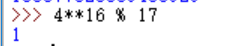### 模反元素

3 和 5 互质，那么 3 的模反元素就是 7，因为 3×7(mod7)=1 $（3 \times 7）\pmod{7} = 1。$

## RSA 操作与原理

RSA 有关参数

P：明文
C：密文

ｅ：公钥
ｄ：私钥


### 生成私钥的过程

1. 随机选定两个大质数 p $p$q$q$

2. 计算 N=p×q $N = p \times q$

3. 计算 φ(N)=(p1)(q1) $\varphi(N) = (p - 1)(q - 1)$

4. 选取正整数 e $e$ ，满足 1 < e$e$ < φ(N) $\varphi(N)$， 且 e $e$φ(N)$\varphi(N)$ 互质

5. 计算 e $e$ 的模反元素 d$d$ ，其中， e $e$d$d$ N $N$ 之间的关系如下：

e×d1(modφ(N))

PeC(modN)

CdP(modN)

### 例 :

=> N=p×q=391 $N = p \times q = 391$

=> 47×d1(modφ(391)) $47 \times d \equiv1\pmod{\varphi (391)}$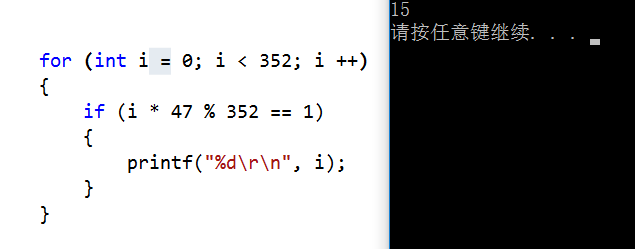#### 加密解密验证

=> C=547(modN)=194$C = {5}^{47}\pmod{ N} =194$

=> P=19415(mod391)=5 $P = {194}^{15} \pmod{391} = 5$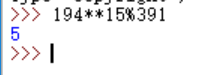### 公式推导

=> Cd(modN)=(Pe)d(modN) ${C}^{d} \pmod{N} = ({P}^{e})^{d}\pmod{N}$
=> (Pe)d(modN)=Pe×d(modN) $({P}^{e})^{d}\pmod{N} = {P}^{e\times d }\pmod{N}$

=> Pe×d(modN)=Pφ(N)×r+1(modN) ${P}^{e\times d }\pmod{N} = {P}^{\varphi(N)\times r + 1} \pmod{N}$
=> Pφ(N)×r+1(modN)=P×Pφ(N)×r(modN) ${P}^{\varphi(N)\times r + 1} \pmod{N} = P \times {P}^{\varphi(N)\times r} \pmod{N}$

=> Pφ(N)×r1(modN) ${P}^{\varphi(N)\times r} \equiv 1\pmod{N}$
=> P×Pφ(N)×r(modN)=P $P \times {P}^{\varphi(N)\times r} \pmod{N} = P$

### RSATool 使用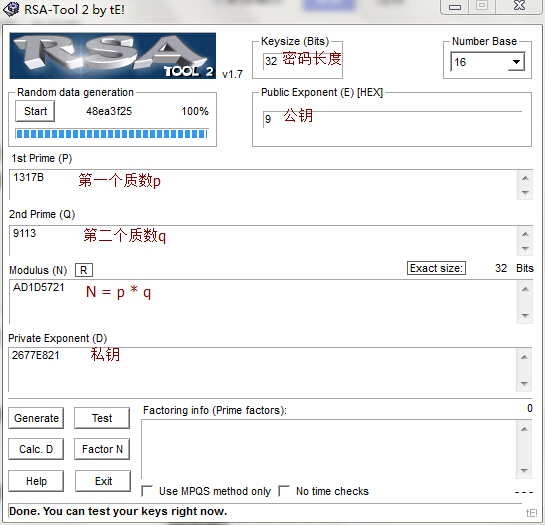#### Factor N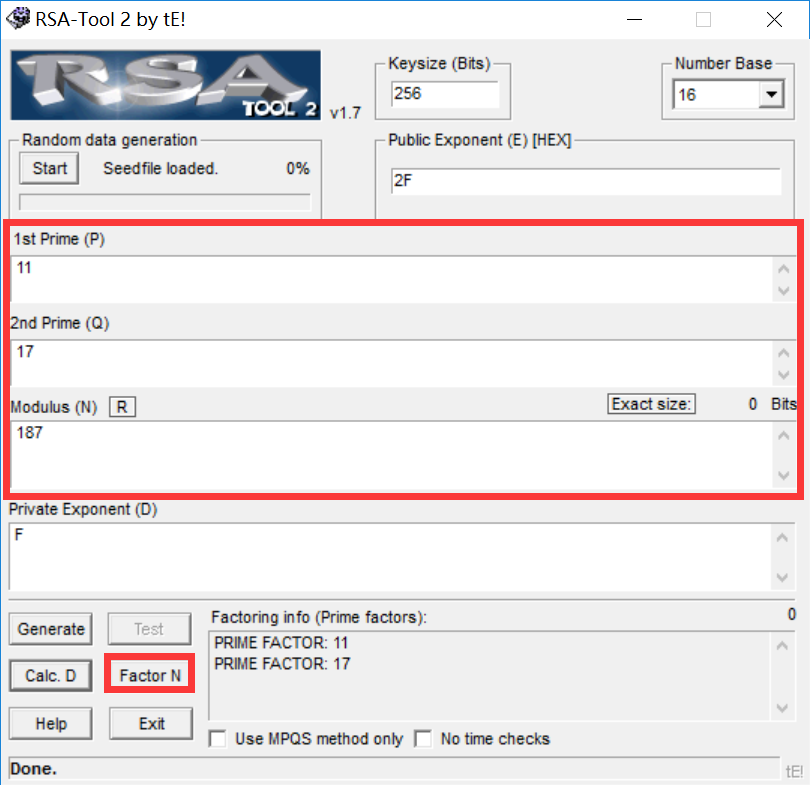#### Calc. D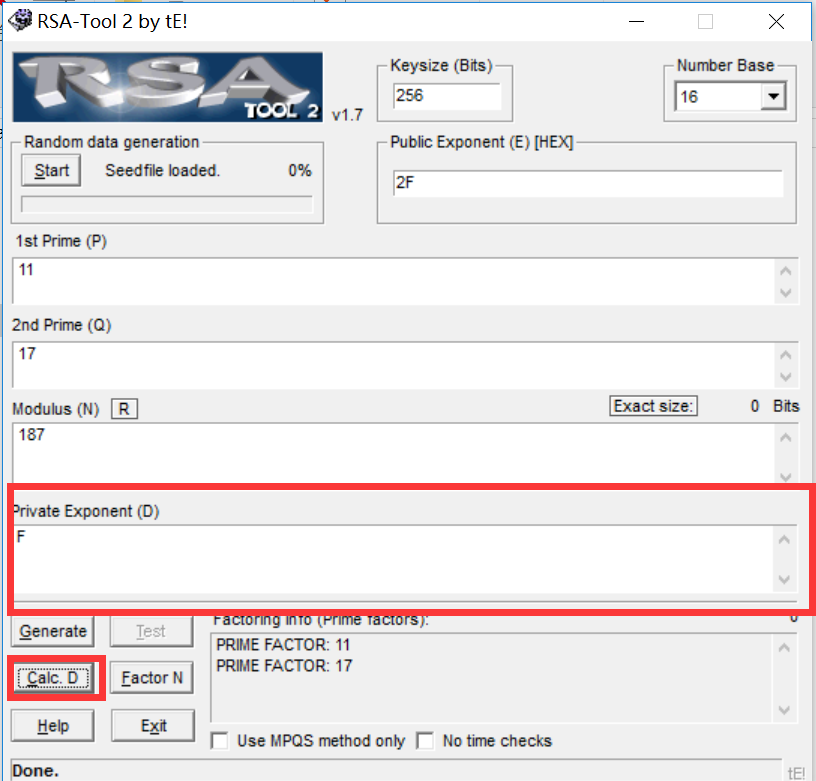E$E$ 是可以随便为一个数，一般都是 10001h。另外，常说的 RSA 有多少位，指的是 N $N$ 有多少位。

### 程序练习

–>程序下载(带大数运算工具及 RSATool)

#### 程序逻辑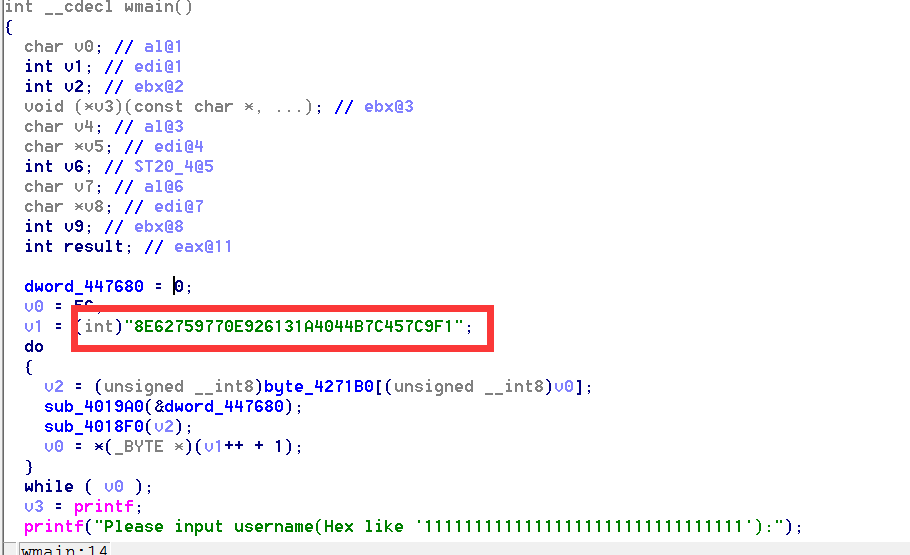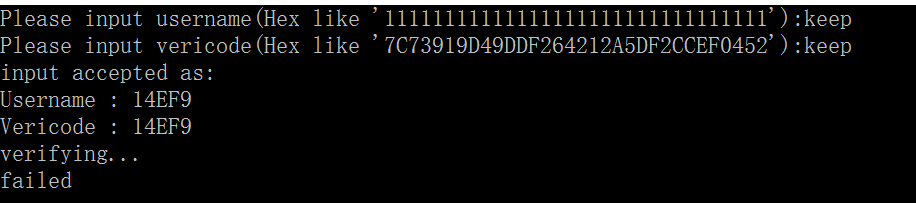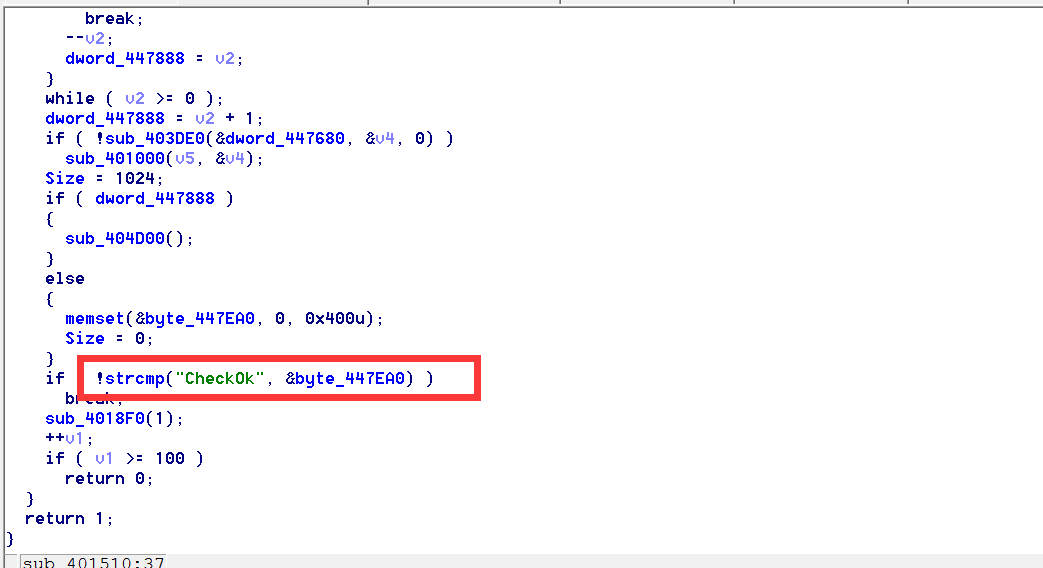#### 破解思路

RSA算法中一般都会使用大数运算，蓝框内显示的是数字的总字节数；红框内的数字从左往右数，为 0x6408FCD6289E9A7A1750886EB8198697。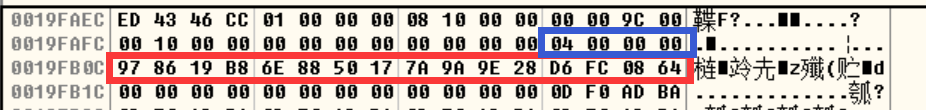e×d1(modφ(N))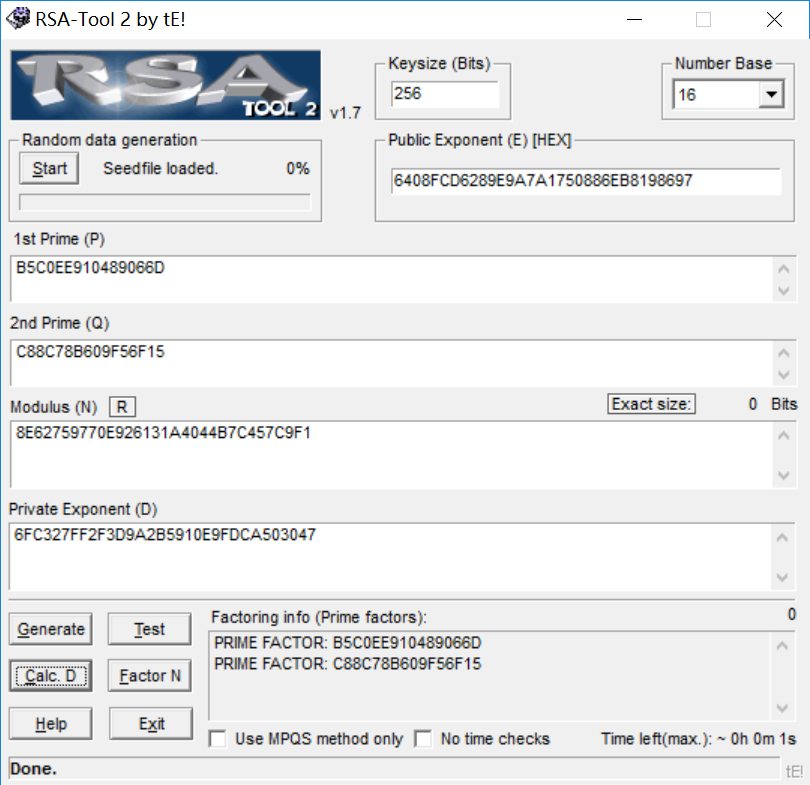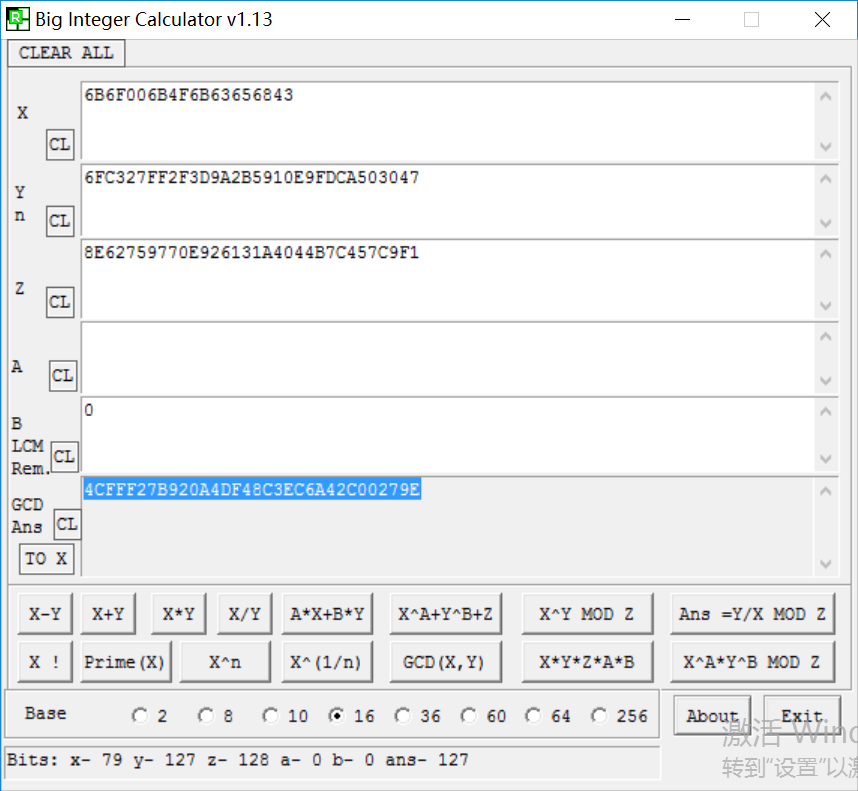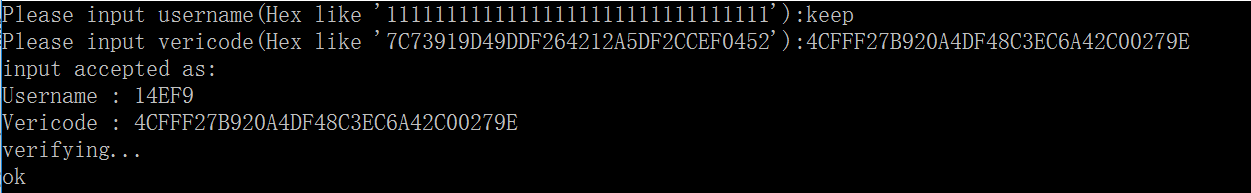01-302万+05-291609
05-29589
01-2751
09-13116
02-243万+
03-1839
10-272980
02-1133
12-2137
10-24115
07-231296
02-1639
10-041万+
06-094401
04-0835
04-1374
©️2020 CSDN 皮肤主题: 编程工作室 设计师:CSDN官方博客